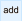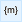﻿ 20-sim webhelp > Language Reference > Keywords > Parameters

# Parameters

Parameters are symbolic representations of numerical quantities which can only be changed after simulation has been stopped. They can therefore be changed in between of simulation runs, or after the user has (temporarily) stopped the simulation.

Parameters must be defined in 20-sim, using the parameters keyword. After the keyword, lines with parameter declarations can be entered. Every line must be finished by a semicolon (;) and may be followed by comment with a description of the parameter. An example is given below:

parameters

real V = 1 {volume, m3};                        // the volume of barrel 1

real hidden D = 0.5 {length, m};                // the length of part 1

real x (range=<-1,1>) = 0.1 {m};                // the position of part x with a min-max range

...

## Types

20-sim currently supports four types of parameters: boolean, integer, real and string. These types must be specified explicitly. The use of the type real is shown in the example above.

## Hidden

To prevent users from inspecting parameters or decreasing the list of parameters, the keyword hidden can be applied. This keyword should follow the data type.

## Global

To use the same parameter in other submodels, the keyword global can be applied. This keyword should follow the data type.

## Parameter Names

Parameter names can consist of characters and underscores. A parameter name must always start with a character. Parameter names are case-sensitive. No reserved words may be used for parameters. If a reserved word is used, an error message will pop-up while checking the model. In the example above the names V and D are used.

## Ranges

Right after a parameter name you can enter annotations for the minimum value, range etc. Examples:

parameters

real V (min=0.1) = 1 {volume, m3};                        // the volume of barrel 1, minimum volume  = 0.1 m3

real R (max=100) = 20 {ohm};                                // the resistance, maximum value  = 100 ohm

real x (range=<-10,10>) = 0.5 {length, m};                // the position of part x, range from -10 to 10 m

real D (readonly=true) = 0.1 {m};                        // the diameter of the the tube, readonly

## Parameter Values

Parameters can be assigned default values. These values can be overridden in the Simulator using the Parameters command.

## Quantity and Unit

If a parameter is known to represent a physical value, you can define the corresponding quantity and unit. 20-sim will use this information to do a unit check on equations. The use of quantities and units is optional. In the example above the quantities volume and length are used with the units m3 and m.

## Comment

If comment is added at the end of the parameter declaration, it is shown in the Variable Chooser to facilitate the selection between variables.

## Example

parameters

real p1 = 12, p2, state = 1.1;

integer i = 10 {length,m};                // vehicle length

integer j = 1 {time,s};

parameters

string filename = 'data.txt';        // This comment appears as a description in the Parameters/Initial values editor.

integer i = 1;                                // Just a parameter

real state;                                        // Initial velocity [m/s]

boolean t = true;                        // T = true

## Tip

 • You can easily enter parameters by using the Add buttonin the Equation Editor Taskbar.
 • You can easily select quantities and units by using the Units buttonof the Equation Editor Taskbar.
 • Use the 20-sim naming convention for parameter names.